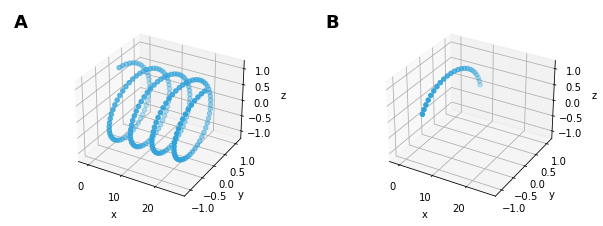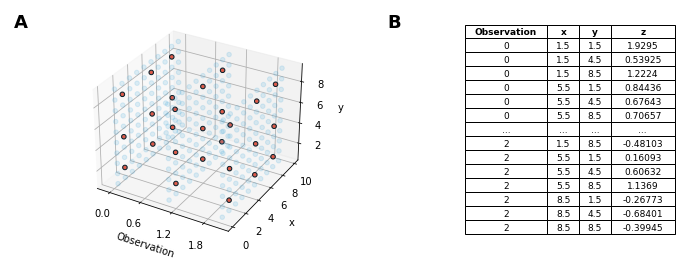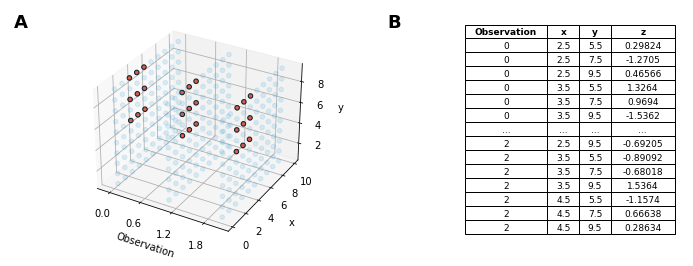# Indexing and Selecting Data#

As explained in the Building composite objects and Dimensioned Containers guides, HoloViews allows building up hierarchical containers that express the natural relationships between data items, in whatever multidimensional space best characterizes the application domain. Once your data is in such containers, individual visualizations are then made by choosing subregions of this multidimensional space, either smaller numeric ranges (as in cropping of photographic images), or lower-dimensional subsets (as in selecting frames from a movie, or a specific movie from a large library), or both (as in selecting a cropped version of a frame from a specific movie from a large library).

In this user guide, we show how to specify such selections, using five different (but related) operations that can act on an element `e`:

Operation

Example syntax

Description

indexing

e[5.5], e[3,5.5]

Selecting a single data value, returning one actual numerical value from the existing data

slice

e[3:5.5], e[3:5.5,0:1]

Selecting a contiguous portion from an Element, returning the same type of Element

sample

e.sample(y=5.5),
e.sample((3,3))

Selecting one or more regularly spaced data values, returning a new type of Element

select

e.select(y=5.5),
e.select(y=(3,5.5))

More verbose notation covering all supported slice and index operations by dimension name.

iloc

e[2, :],
e[2:5, :]

Indexes and slices by row and column tabular index supporting integer indexes, slices, lists and boolean indices.

These operations are all concerned with selecting some subset of the data values, without combining across data values (e.g. averaging) or otherwise transforming the actual data. In the Tabular Data user guide we will look at additional operations on the data that reduce, summarize, or transform the data in other ways, in addition to the selections covered here.

We’ll be going through each operation in detail and provide a visual illustration to help make the semantics of each operation clear. This user guide assumes that you are familiar with continuous and discrete coordinate systems, so please review our Continuous Coordinates guide if you have not done so already.

```import numpy as np
import holoviews as hv
from holoviews import opts

hv.extension('bokeh', 'matplotlib')

opts.defaults(
opts.Bounds(line_width=2, color='red', axiswise=True),
opts.Image(cmap='Blues'),
opts.Text(text_font_size='16pt'), opts.Scatter(size=5))
```## Indexing and slicing Elements#

In the Dimensioned Containers guide we saw examples of how to select individual elements embedded in a multi-dimensional space. The Continuous Coordinates user guide covered slicing and indexing in Elements representing continuous coordinate coordinate systems such as `Image` types. Here we’ll be going through each operation in full detail, providing a visual illustration to help make the semantics of each operation clear.

How the `Element` may be indexed depends on the key dimensions (or `kdims`) of the `Element`. It is thus important to consider the nature and dimensionality of your data when choosing the `Element` type for it.

### 1D Elements: Slicing and indexing#

Certain Chart elements support both single-dimensional indexing and slicing: `Scatter`, `Curve`, `Histogram`, and `ErrorBars`. Here we’ll look at how we can easily slice a `Histogram` to select a subregion of it:

```np.random.seed(42)
edges, data = np.histogram(np.random.randn(100))
hist = hv.Histogram((edges, data))
subregion = hist[0:1]
hist * subregion
```

The two bins in a different color show the selected region, overlaid on top of the full histogram. We can also access the value for a specific bin in the `Histogram`. A continuous-valued index that falls inside a particular bin will return the corresponding value or frequency.

```hist[0.25], hist[0.5], hist[0.55]
```
```(21, 21, 11)
```

We can slice a `Curve` the same way:

```xs = np.linspace(0, np.pi*2, 21)
curve = hv.Curve((xs, np.sin(xs)))
subregion = curve[np.pi/2:np.pi*1.5]
curve * subregion * hv.Scatter(curve)
```

Here again the region in a different color is the specified subregion. We’ve also marked each discrete point with a dot using the `Scatter` `Element`. As before we can also get the value for a specific sample point; whatever x-index is provided will snap to the closest sample point and return the dependent value:

```curve[4.05], curve[4.1], curve[4.17], curve[4.3]
```
```(-0.8090169943749475,
-0.8090169943749475,
-0.8090169943749475,
-0.9510565162951535)
```

It is important to note that an index (or a list of indices, as for the 2D and 3D cases below) will always return the raw indexed (dependent) value, i.e. a number. A slice (indicated with `:`), on the other hand, will retain the Element type even in cases where the plot might not be useful, such as having only a single value, two values, or no value at all in that range:

```curve[4:4.5]
```

### 2D and 3D Elements: slicing#

For data defined in a 2D space, there are 2D equivalents of the 1D `Curve` and `Scatter` types. `Points`, for example, can be thought of as a number of points in a 2D space.

```r = np.arange(0, 1, 0.005)
xs, ys = (r * fn(85*np.pi*r) for fn in (np.cos, np.sin))
paths = hv.Points((xs, ys))
paths + paths[0:1, 0:1]
```

However, indexing is not supported in this space, because there could be many possible points near a given set of coordinates, and finding the nearest one would require a search across potentially incommensurable dimensions, which is poorly defined and difficult to support.

Slicing in 3D works much like slicing in 2D, but indexing is not supported for the same reason as in 2D:

```xs = np.linspace(0, np.pi*8, 201)
scatter = hv.Scatter3D((xs, np.sin(xs), np.cos(xs)))
layout = scatter + scatter[5:10, :, 0:]
hv.output(layout, backend='matplotlib')
```### 2D Raster and Image: slicing and indexing#

Raster and the various other image-like objects (Images, RGB, HSV, etc.) can all be sliced and indexed, as can Surface, because they all have an underlying regular grid of key dimension values:

```np.random.seed(0)
extents = (0, 0, 10, 10)
img = hv.Image(np.random.rand(10, 10), bounds=extents)
img_slice = img[1:9,4:5]
box = hv.Bounds((1,4,9,5))
img*box + img_slice
```
```img[4.2,4.2], img[4.3,4.2], img[5.0,4.2]
```
```(0.2088767560948347, 0.2088767560948347, 0.16130951788499626)
```

## Tabular indexing and slicing#

While most indexing in HoloViews works by selecting the values along a dimension it is also frequently useful to index and slice using integer row and column indices. For this purpose most HoloViews objects have a `.iloc` indexing interface (mirroring the pandas API), which supports all the usual indexing semantics. Supported iloc arguments include:

• An integer e.g. 5

• A list or array of integers [4, 3, 0]

• A slice object with ints 1:7

• A boolean array

### Indexing#

In this way we can for example select the x- and y-values in the 8th row of our `Curve`:

```xs = np.linspace(0, np.pi*2, 21)
curve = hv.Curve((xs, np.sin(xs)))
print('x: %s, y: %s' % (curve.iloc[8, 0], curve.iloc[8, 1]))
curve * hv.Scatter(curve.iloc)
```
```x: 2.5132741228718345, y: 0.5877852522924731
```

### Slicing#

Alternatively we can select every second sample between indices 5 and 16 of a `Curve`:

```curve + curve.iloc[5:16:2]
```

### Lists of integers and boolean indices#

Finally we may also pass a list of the integer samples to select, or use boolean indices. This mode of indexing can be very useful for randomly sampling an Element or picking a specific set of rows or (columns):

```curve.iloc[[0, 5, 10, 15, 20]] + curve.iloc[xs>3]
```

## Sampling#

Sampling is essentially a process of indexing an Element at multiple index locations, and collecting the results. Thus any Element that can be indexed can also be sampled. Compared to regular indexing, sampling is different in that multiple indices may be supplied at the same time. Also, indexing will only return the value at that location, whereas the return type from a sampling operation is another `Element` type, usually either a `Table` or a `Curve`, to allow both key and value dimensions to be returned.

### Sampling Elements#

Sampling can use either an explicit list of indexes, or pass an index value for each dimension keyword argument.

We’ll start by taking a single sample of an Image object, to make clear how sampling and indexing are similar operations yet different in their results:

```img_coords = hv.Points(img, extents=extents)
labeled_img = img * img_coords * hv.Points([img.closest([(4.1,4.3)])]).opts(color='r')
img + labeled_img + img.sample([(4.1,4.3)])
```
```img[4.1,4.3]
```
```0.2088767560948347
```

Here, the output of the indexing operation is the value (0.20887675609483469) from the location closest to the specified indexes, whereas `.sample()` returns a Table that lists both the coordinates and the value, and slicing (in previous section) returns an Element of the same type, not a Table.

Next we can try sampling along only one Dimension on our 2D Image, leaving us with a 1D Element (in this case a `Curve`):

```sampled = img.sample(y=5)
labeled_img = img * img_coords * hv.Points(zip(sampled['x'], [img.closest(y=5)]*10))
img + labeled_img + sampled
```

Sampling works on any regularly sampled Element type. For example, we can select multiple samples along the x-axis of a Curve.

```xs = np.arange(10)
samples = [2, 4, 6, 8]
curve = hv.Curve(zip(xs, np.sin(xs)))
curve_samples = hv.Scatter(zip(xs,  * 10)) * hv.Scatter(zip(samples, *len(samples)))
curve + curve_samples + curve.sample(samples)
```

### Sampling HoloMaps#

Sampling is often useful when you have more data than you wish to visualize or analyze at one time. First, let’s create a HoloMap containing a number of observations of some noisy data.

```obs_hmap = hv.HoloMap({i: hv.Image(np.random.randn(10, 10), bounds=extents)
for i in range(3)}, kdims='Observation')
```

A `HoloMap` may not be sampled directly, instead we can use the `.apply` method to sample each element in the HoloMap and consequently use the `.collapse` method to produce a single `Dataset`. In this case we’ll take 3x3 subsamples of each of the Images:

```hv.output(backend='matplotlib', size=120)

sample_style = dict(edgecolors='k', alpha=1)
all_samples = obs_hmap.collapse().to.scatter3d().opts(alpha=0.15, xticks=4)
sampled = obs_hmap.apply.sample((3,3)).collapse()
subsamples = sampled.to.scatter3d().opts(**sample_style)
all_samples * subsamples + hv.Table(sampled)
```By supplying bounds in as a (left, bottom, right, top) tuple we can also sample a subregion of our images:

```sampled = obs_hmap.apply.sample((3,3), bounds=(2,5,5,10)).collapse()
subsamples = sampled.to.scatter3d().opts(xticks=4, **sample_style)
all_samples * subsamples + hv.Table(sampled)
```Since this kind of sampling is only well supported for continuous coordinate systems, we can only apply this kind of sampling to Image types for now.

### Sampling Charts#

Sampling Chart-type Elements like Curve, Scatter, Histogram is only supported by providing an explicit list of samples, since those Elements have no underlying regular grid.

```hv.output(backend='bokeh')

xs = np.arange(10)
extents = (0, 0, 2, 10)
curve = hv.HoloMap({(i) : hv.Curve(zip(xs, np.sin(xs)*i))
for i in np.linspace(0.5, 1.5, 3)},
kdims='Observation')
all_samples = curve.collapse().to.points()
sampled = curve.apply.sample([0, 2, 4, 6, 8]).collapse()
sample_points = sampled.to.points(extents=extents)
sampling = all_samples * sample_points.opts(color='red')
sampling + hv.Table(sampled)
```

These tools should help you index, slice, sample, and select your data with ease. The Tabular Data guide explains how to do other types of operations, such as averaging and other reduction operations.

This web page was generated from a Jupyter notebook and not all interactivity will work on this website. Right click to download and run locally for full Python-backed interactivity.